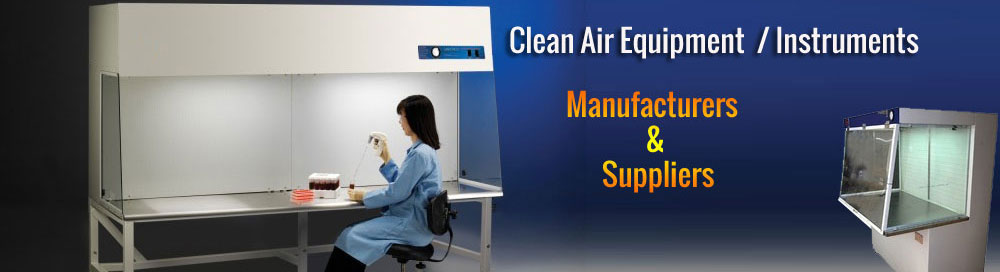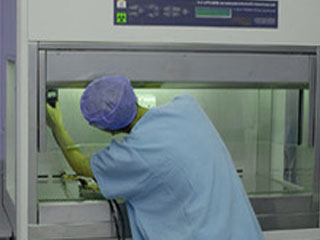Velocity Uniformity Test
•These tests are performed to determine the average filter face velocity and uniformity, and / or the average room airflow velocity and uniformity within a cleanroom. Airflow velocity (meters per second or feet per minute) is a measurement of the speed of the airflow. An average velocity is calculated by dividing the cleanroom / filter area into equal grids and taking the airflow velocity in the center of each of these grids. The average airflow velocity is calculated by dividing the total of the airflow grid velocities by the number of readings taken.